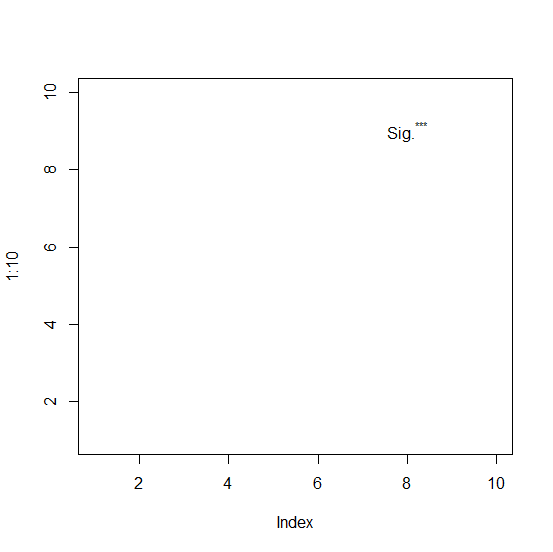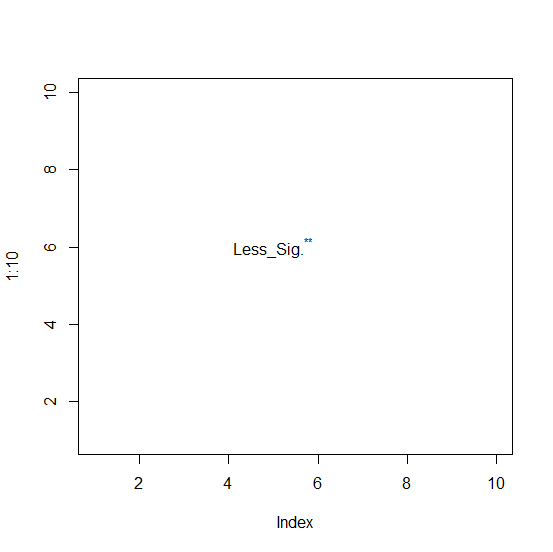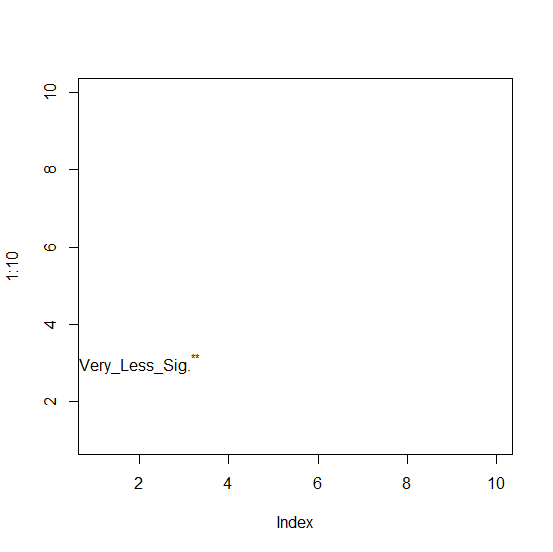# How to display star/asterisk sign (*) inside a base R plot?

To display characters inside a base R plot we can simply use text function with expression and if we want to display an asterisk then we need to put the asterisk within double quotes. For example, if we want to display three stars then only expression(paste("***"))) should be used. Check out the below examples to understand how it works.

## Example1

> plot(1:10,type="n")
> text(8,9,expression(paste(Sig.^"***")))

## Output## Example2

> plot(1:10,type="n")
> text(5,6,expression(paste(Less_Sig.^"**")))

## Output## Example3

> plot(1:10,type="n")
> text(2,3,expression(paste(Very_Less_Sig.^"**")))

## Output